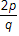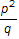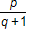# Study Guide

## Field 212: Multi-Subject: Teachers of Early Childhood (Birth–Grade 2) Part Two: Mathematics

### Sample Selected-Response Questions

The following reference material will be available to you during the test:

#### Competency 0001 Number and Operations

1. Given that p and q are distinct prime numbers greater than 2, which of the following could be a whole number?

1.2.3.4.Correct Response: D. This question requires the examinee to apply number theory concepts. Prime numbers are numbers whose only factors are the number 1 and the number itself. Choose prime numbers and test them in each of the expressions. For example, if  p equals 7  and  q equals 7  then the only expression that represents a whole number is choice D: seven plus one over five minus one equals 2.

#### Competency 0002 Operations and Algebraic Thinking

2. Use the diagram below to answer the question that follows.An artist creates a cube using a piece of clay. The artist then cuts the cube in half to make two congruent rectangular solids, as shown in the diagram. Which of the following is the ratio of the surface area of the original cube to the total surface area of the resulting rectangular solids?

1.  1 to 2
2.  2 to 3
3.  3 to 4
4.  5 to 6
Correct Response: C. This question requires the examinee to solve unit-rate problems including those involving ratios of areas. Since the original solid is a cube, its surface area is 6 times the area of one face. Suppose the area of one face is 1 square unit, then the original surface area is 6 square units. By cutting the cube in half, the artist has created two new surfaces, each with area 1 square unit, and kept the other surfaces the same. Thus the total area of the two solids is 8 square units. The ratio of the original surface area to the new total surface area is  6 to 8  or  3 to 4.

#### Competency 0003 Measurement, Geometry, and Data

3. Use the diagram below to answer the question that follows.

river with several points labeled. point a is on the bank point b is on the bank of the river on the southern side the distance between a and b is fifty feet c is directly across the river on the northern bank there are line segments connecting a b and c. point d is on the bank to the right one hundred feet of point c point e is thirty feet to the left of point b there are line segments drawn to connect d e and a. the line segments make triangle a c d with being a line segement through the triangle and side a d being the hypotenuse

The diagram represents a method for estimating  B C,  the width of a river. Given that  A B equals 50 feet,   C D equals 100 feet  and  B E equals 30 feet,  which of the following is the best estimate for  B C ?

1. 60 feet
2. 80 feet
3. 117 feet
4. 167 feet
Correct Response: C. This question requires the examinee to solve problems involving similarity.  Triangle A B E  is similar to  Triangle A C D  because their corresponding angles are congruent. Therefore the corresponding sides of the two triangles are proportional: fifty over a c equals thirty over one hundred, and  30 left paren A C right paren equals 5000.  To the nearest foot,  A C is 167,  so   B C is 167 minus 50  or 117 feet.

#### Competency 0004 Instruction in Mathematics

4. Use the diagram below to answer the question that follows.A teacher places a set of blocks on a table and asks a child how many blocks there are. The child points to each block while saying the numbers in the proper sequence from one through five. When asked again how many blocks there are, the child responds by counting the number of blocks again. Given this evidence, which question could the teacher ask to help the child connect counting to cardinality?

1. How many cones, boxes, and balls are there?
2. How is the last number name you said related to the number of blocks?
3. How many different types of shapes are there?
4. How is the first block counted similar to the last block counted?